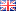External Status LED's for Raspberry Pi (without GPIO)Introduction
The Raspberry Pi is a great device for all kinds of applications. For some of these applications you want to build the Rpi in a housing where the status LEDs are no longer visible. I like to be able to see the LED's for some basic diagnostics.

My solution:
• Low cost. Componects can be bought for less than € 1,-
• No connection to GPIO needed.
• No code needed. Works without any software.

Here i will explain a simple solution to extend the status LED's to the outside of any enclosure. This solution requires soldering on the PCB of the Rpi, but only in a very simple way. If you have soldered on a PCB before, you can easily do this with risking damaging your Pri.

The Rpi have many test pad's that can be used for all kinds of diagnostics. There are also pad's connected to the status LED's of the Rpi PCB, and we can tap into these. The problem is they will not directly give a suitable voltage (or current) to directly attach a LED. I would much rather have seen these test pads connected differently but we need to work with what we have.

The test pad's give about 3.3V when the LED's is of, and down to 2V when the LED is on. We need to translate this to 0V and 5V so we can sent it to a LED. In basic electronics there is a very simple solution for this. Its called a comparator. Have a look at the schematic below:

Schematics

How does a comparator work?
A comparator can be build with a simple OpAmp. A OpAmp has a positive input and negative input. Putting a voltage on the positive input will raise the output voltage. Putting a voltage on the negative input wil lower it. A OpAmp (operational amplifier) wil also amplify the input. You can set is to 0, to 10x, to 1000x, etc. By default the gain is extremely high. For example 100.000 times or even much higher.

In a comparator we can use both characteristics to our advantage. If we compare the voltage of the negative input to our positive input we can calculate the output.

For example: -5V + 6V = 1V. Simple right? Now if we multiply this by 100.000 we get a output voltage of 100.000V. Off course this if far from possible, so what happens in the real world? The opamp "clips" to the available power supply (minus a small voltage needed by the OpAmp to work) As a opamp has a positive power supply (Vcc+) and a negative power supply (Vcc-) the opamp will clip to Vcc+ in this example.

So a comparator can compare a reference voltage to a measured voltage an tell you which one is higher. If you use a positive power supply only(Vcc+) of 5V (Vcc- connected to GND) and you have a 0,001V (1mV) difference between your voltage reference and the measured voltage, the output of the comparator will be either 0V or 5V. This is great for controlling a LED. The average power output of a opamp is enough to supply enough power to a few LED's

The PCB with comparator and LED's.

Back to the test pad's. We know ther voltages are approx 3,3V with the LED's off, and 2V with the LED on. If we compare this to 2,65V (the number between 2 and 3,3) the comparator can easily tell if the LED is on or off.

So we need a reference voltage (Vref) for the comparator to work with. Actually anything below 3,3V and above 2V will do, but these voltages may vary a little bit. A voltage divider with resistors is the most simple way to do this.

2 resistors of the same value connected between 5V (Vcc) and GND will give 2,5V, This is close enough so this will work fine in most cases. If 5V however is actually 4,5V the output of the divider will be 2,25. If you use the populair E12 resistor series you can have a 10% error in the resistor value. This can make your Vref drop below 2V. Therefore i prefer setting the Vref manually with a precision trim potentiometer. I most cases however 2 resistors will work just as good.